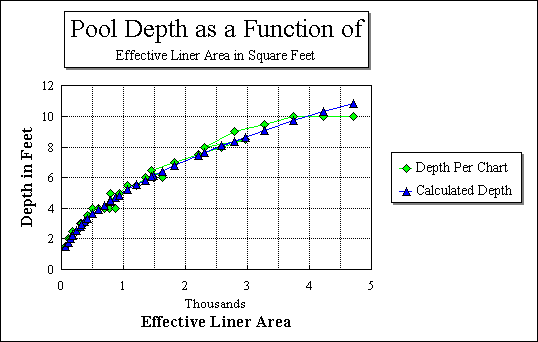##The liner optimizer is a way to design a RECTANGULAR or SQUARE pool that will hold the maximum amount of water for a given liner size. The concept and methodology are those of Greg Bickal, who points out the following:

1. For maximum water volume plant shelves are not allowed.

2. While a round bottom would maximize volume, round bottoms are impractical if you plan on having plants sitting on plant stands. However, a considerable amout of volume can be gained by "softening" (Greg's term) the corners where the sides meet the bottom, See Greg's website for an explanation and diagram. This "corner softening" has been built into the model, so that you will not be able to simply multiply length by width by depth to get volume as the bottom is partially "rounded".

The model assumes that the optimal depth and pool size for a given rectangular pool is a function of the effective area of the liner, and is estimated using the following formula:

Depth = Ö (Area + 25) /40

The graph below compares the values from Greg Bickal's chart to the values provided by the model. Differences are nominal and are the result of different rounding techniques.